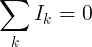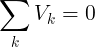# Kirchhoff's laws (KVL / KCL)

Kirchhoff's current law and voltage law, defined by Gustav Kirchhoff, describe the relation of values of currents that flow through a junction point and voltages in a an electrical circuit loop, in an electrical circuit.

## Kirchhoff's Laws introduction

Kirchhoff’s rules, two statements about multi-loop electric circuits that embody the laws of conservation of electric charge and energy and that are used to determine the value of the electric current in each branch of the circuit.

The first rule, the junction theorem, states that the sum of the currents into a specific junction in the circuit equals the sum of the currents out of the same junction. Electric charge is conserved: it does not suddenly appear or disappear; it does not pile up at one point and thin out at another.

The second rule, the loop equation, states that around each loop in an electric circuit the sum of the emf’s (electromotive forces, or voltages, of energy sources such as batteries and generators) is equal to the sum of the potential drops, or voltages across each of the resistances, in the same loop. All the energy imparted by the energy sources to the charged particles that carry the current is just equivalent to that lost by the charge carriers in useful work and heat dissipation around each loop of the circuit.

## Kirchhoff's Current Law (KCL)

This is Kirchhoff's first law.

The sum of all currents that enter an electrical circuit junction is 0. The currents enter the junction have positive sign and the currents that leave the junction have a negative sign:Another way to look at this law is that the sum of currents that enter a junction is equal to the sum of currents that leave the junction:

#### KCL example

I1 and I2 enter the junction

I3 leave the junction

I1=2A, I2=3A, I3=-1A, I4= ?

Solution:

Ik = I1+I2+I3+I4 = 0

I4 = -I1 - I2 - I3 = -2A - 3A - (-1A) = -4A

Since I4 is negative, it leaves the junction.

## Kirchhoff's Voltage Law (KVL)

This is Kirchhoff's second law.

The sum of all voltages or potential differences in an electrical circuit loop is 0.#### KVL example

VS = 12V, VR1 = -4V, VR2 = -3V

VR3 = ?

Solution:

Vk = VS + VR1 + VR2 + VR3= 0

VR3 = -VS - VR1 - VR2 = -12V+4V+3V = -5V

The voltage sign (+/-) is the direction of the potential difference.

Currently, we have around 1975 calculators, conversion tables and usefull online tools and software features for students, teaching and teachers, designers and simply for everyone.